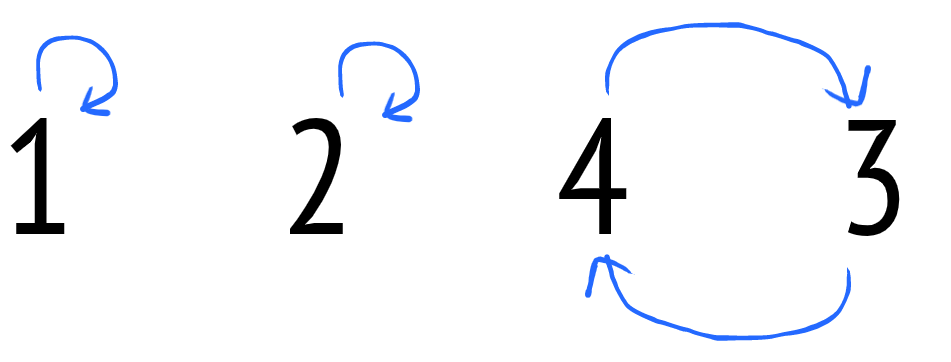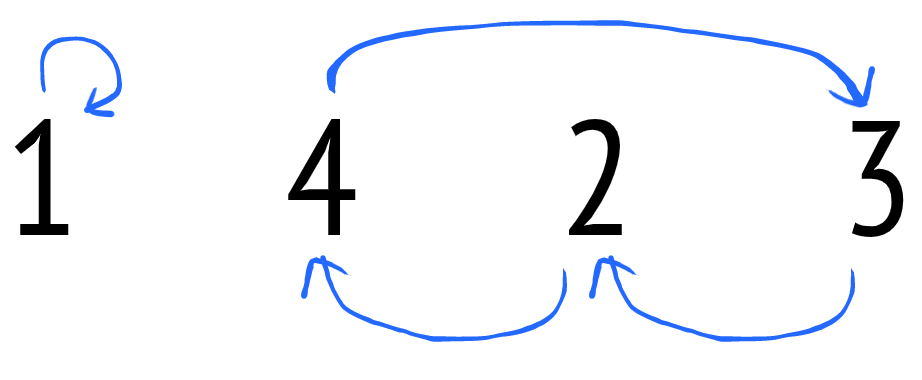# Lily's Homework

See the original problem on HackerRank.

## Solutions

The sum is minimal when the array is sorted. Both descending and ascending order will yield the minimum sum of the absolute difference of adjacent elements. We need to count the number of swaps in both cases.

To find the number of swaps we have to find the number of cycles in the array. For instance, given the following array:

 1  1 2 4 3 

We can construct a directed graph by making each value of the array point to its sorted position:

• $$1 \rightarrow 1$$
• $$2 \rightarrow 2$$
• $$4 \rightarrow 3$$
• $$3 \rightarrow 4$$

Visually:Other examples:Intuitively, a cycle is a directed path that starts and finishes on the same value. The length of a cycle is the number of arrows the cycle is made from.

You can see that given a cycle of length $$l$$, making all its values reach their correct order require $$l-1$$ swaps. Try that yourself.

Thus, the total number of swaps required to sort the array is given by summing up all the cycle “resolutions”.

One possible solution to the original problem consists in calculating the number of swaps for both the array and the reversed array, and then returning the minimum value between the two.

A map is used as a support data structure. Alternatively, an array of pairs is very common in this kind of problems (left as an exercise).

Roughly, the idea consists in sorting a copy of input array and filling up the map with the input element positions.

Then we compare each element of the input array with the sorted array. If any mismatch is found, we have to swap the element out of position with the “expected” one.

For example:

 1  1 2 4 3 

1 and 2 are found in their final position. Instead, 4 is not and then it gets swapped with 3.

The position table is updated as well, just by changing the position of the element out of order to the expected element position.

In the example above, the position table is initialized as follows:

 1 2 3 4  1 -> 0 2 -> 1 3 -> 3 4 -> 2 

When 4 and 3 are swapped, the position of 4 is set to 3.

Another example:

 1  2 5 3 1 

The position table:

 1 2 3 4  1 -> 3 2 -> 0 3 -> 2 5 -> 1 

The first iteration finds that 2 is out of order. It gets swapped with 1 and the position of 2 is updated. So we have:

 1  1 5 3 2 

And the position table:

 1 2 3 4  1 -> 3 (not used anymore) 2 -> 3 3 -> 2 5 -> 1 

The second iteration find that 5 is out of order and it gets swapped with 2 (found thanks to having updated the position table before):

 1  1 2 3 5 

And the position table:

 1 2 3 4  1 -> 3 (not used anymore) 2 -> 3 (not used anymore) 3 -> 2 5 -> 3 

In the third and fourth iterations, the algorithm does nothing.

At the end of the main loop, we have an interesting side effect: each element position is the last one of its cycle, this makes a sort of “grouping by cycle”.

Here is a possible C++ implementation:

  1 2 3 4 5 6 7 8 9 10 11 12 13 14 15 16 17 18 19 20 21 22 23 24 25 26 27 28 29 30 31 32 33 34  int swaps(vector& v) { auto sorted = v; sort(begin(sorted), end(sorted)); auto n = v.size(); map m; for (auto i=0u; i> n; vector v(n); copy_n(istream_iterator(cin), n, begin(v)); vector reversed(rbegin(v), rend(v)); cout << min(swaps(v), swaps(reversed)); } 

The cost is, clearly, $$O(N \cdot logN)$$.